Maths-
General
Easy

Question

# For the ellipse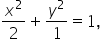the foci are

## ( ±1, 0)    (0, ± 1)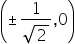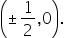Hint:

## The correct answer is: ( ±1, 0)

### Given : Equation of ellipseCenter of ellipse = (h, k) = (0,0)Eccentricity (e) =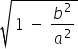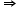e =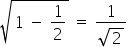Foci = ( h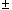ae , k)where a =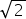Foci = ( 0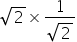, 0)Foci = ( 0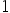, 0)Foci = (, 0)#### With Turito Foundation.#### Get an Expert Advice From Turito.